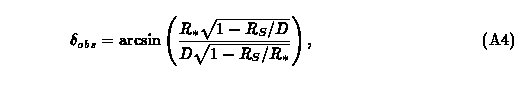from Nemiroff, R. J., American Journal of Physics, 61, 619 (1993)

## APPENDIX

What is the apparent angular size of a sphere of mass M (corresponding to Schwarzschild radius R_S), radius R_*, at distance D? This extremely simple and beautiful problem is important to observable aspects of high gravity environments. The impact parameter at infinity, here labeled b, is a constant along the trajectory of the photon.  At any radius r from a sphere with mass M with Schwarzschild R_S on the photon's trajectory, this constant is equal towhere delta is the angle the photon's velocity makes with the radial direction. For a lens large enough not to exhibit a photon sphere (R_* > 1.5 R_S), the limb of the source will be seen when a photon leaving the source tangentially grazing its surface, such that r = R_* and delta = pi/2. ThenThis photon will reach the observer with \$r = D\$ and angle to the center of the lens of delta_obs such thatEquating Eqs. (A2) and (A3) and solving for delta_obs yieldswhich is the apparent size of the lens.

When the lens is compact enough to be surrounded by a photon sphere, one can simply replace R_* in the above expression with 1.5 R_S, as these photons now define the apparent size of the lens. The result isBe careful to assign the correct quadrant to the result. If R_* < 1.5 R_S, then delta_obs will be greater than pi/2.

References
Back to Virtual Trips to Black Holes and Neutron Stars Page